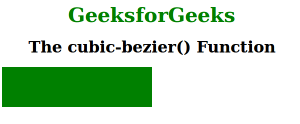# CSS | cubic-bezier() Function

• Last Updated : 30 Jul, 2021

The cubic-bezier() function is an inbuilt function in CSS which is used to defines a Cubic Bezier curve.
A Bezier curve is a mathematically defined curve used in two-dimensional graphic applications like abode illustrator, inkscape etc.The curve is defined by four points: the initial position and the terminating position i.e P0 and P3 respectively (which are called “anchors”) and two separate middle points i.e P1 and P2(which are called “handles”) in our example.Bezier curves are frequently used in computer graphics, animation, modeling etc.
Syntax:

`cubic-bezier( x1, y1, x2, y2 )`

Parameters: This function accepts four parameter which is mandatory. It contains a numeric value and the value of x1 and x2 lies between 0 to 1.
Below program illustrates the cubic-bezier() function in CSS:
Program:

Attention reader! Don’t stop learning now. Get hold of all the important HTML concepts with the Web Design for Beginners | HTML  course.

## html

 ```<``html``>``    ``<``head``>``        ``<``title``>cubic-bezier function``        ``<``style``>``            ``.geeks {``                ``width: 150px;``                ``height: 80px;``                ``background: green;``                ``transition: width 5s;``                ``transition-timing-function: cubic-bezier(0.3, 0.7, 1.0, 0.1);``            ``}``            ` `            ``div:hover {``                ``width:300px;``            ``}``            ``.gfg {``                ``font-size:40px;``                ``font-weight:bold;``                ``color:green;``                ``text-align:center;``            ``}``            ``h1 {``                ``text-align:center;``            ``}``        ````    ````    ``<``body``>``        ``<``div` `class` `= ``"gfg"``>GeeksforGeeks``        ``<``h1``>The cubic-bezier() Function``        ``<``div` `class` `= ``"geeks"``>``    `````

Output:Supported Browser: